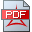1. A mathematical framework for an integrated gravity and quantum modelA mathematical framework for an integrated gravity and quantum model, 2011-2017

2. An alternative QED model based on a quantum state Hilbert space H(-1/2) enabling also a quantum gravity model

3. An alternative quantum ground state energy model based on a quantum state Hilbert space H(-1/2) enabling also a quantum gravity model